Single Hydraulic Cylinder Simulation

This example shows how to use Simulink® to model a hydraulic cylinder. You can apply these concepts to applications where you need to model hydraulic behavior. See two related examples that use the same basic components: four cylinder model and two cylinder model with load constraints.

• Note: This is a basic hydraulics example. You can more easily build hydraulic and automotive models using Simscape™ Driveline™ and Simscape Fluids™.

• Simscape Fluids provides component libraries for modeling and simulating fluid systems. It includes models of pumps, valves, actuators, pipelines, and heat exchangers. You can use these components to develop fluid power systems such as front-loader, power steering, and landing gear actuation systems. Engine cooling and fuel supply systems can also be developed with Simscape Fluids. You can integrate mechanical, electrical, thermal, and other systems using components available in the Simscape product family.

• Simscape Driveline provides component libraries for modeling and simulating one-dimensional mechanical systems. It includes models of rotational and translational components, such as worm gears, planetary gears, lead screws, and clutches. You can use these components to model the transmission of mechanical power in helicopter drivetrains, industrial machinery, vehicle powertrains, and other applications. Automotive components, such as engines, tires, transmissions, and torque converters, are also included.

Analysis and Physics of the Model

Figure 1 shows a schematic diagram of the basic model. The model directs the pump flow, Q, to supply pressure, p1, from which laminar flow, q1ex, leaks to exhaust. The control valve for the piston/cylinder assembly is modeled as turbulent flow through a variable-area orifice. Its flow, q12, leads to intermediate pressure, p2, which undergoes a subsequent pressure drop in the line connecting it to the actuator cylinder. The cylinder pressure, p3, moves the piston against a spring load, resulting in position x.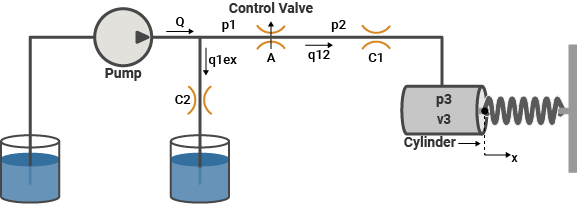Figure 1: Schematic diagram of the basic hydraulic system

At the pump output, the flow is split between leakage and flow to the control valve. We model the leakage, q1ex, as laminar flow (see Equation Block 1).

Equation Block 1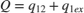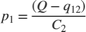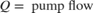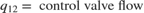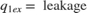We modeled turbulent flow through the control valve with the orifice equation. The sign and absolute value functions accommodate flow in either direction (see Equation Block 2).

Equation Block 2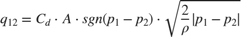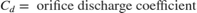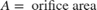The fluid within the cylinder pressurizes due to this flow, q12 = q23, minus the compliance of the piston motion. We also modeled fluid compressibility in this case (see Equation Block 3).

Equation Block 3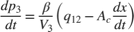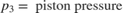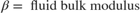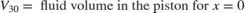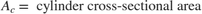We neglected the piston and spring masses because of the large hydraulic forces. We completed the system of equations by differentiating this relationship and incorporating the pressure drop between p2 and p3. Equation Block 3 models laminar flow in the line from the valve to the actuator. Equation block 4 gives the force balance at the piston.

Equation Block 4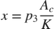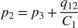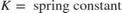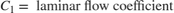Modeling

Figure 2 shows the top level diagram of the model. The pump flow and the control valve orifice area are simulation inputs. The model is organized as two subsystems: the 'Pump' and the 'Valve/Cylinder/Piston/Spring Assembly'.

Opening the Model and Running the Simulation

To open this model, type sldemo_hydcyl at MATLAB® terminal (click on the hyperlink if you are using MATLAB Help). Press the "Play" button on the model toolbar to run the simulation.

The model logs relevant data to MATLAB workspace, into the Simulink.SimulationOutput object out. The signal logging data is stored in the out object, in a structure called sldemo_hydcyl_output. Logged signals have a blue indicator (see the model). For more information, see View and Access Signal Logging Data.Figure 2: Single cylinder model and simulation results

'Pump' Subsystem

Right-click the Pump masked subsystem and select Mask > Look Under Mask. The pump model computes the supply pressure as a function of the pump flow and the load (output) flow (Figure 3). Qpump is the pump flow data (saved in the model workspace). A matrix with column vectors of time points and the corresponding flow rates [T, Q] specifies the flow data. The model calculates pressure p1 as indicated in Equation Block 1. Because Qout = q12 is a direct function of p1 (via the control valve), an algebraic loop is formed. An estimate of the initial value, p10, enables a more efficient solution.Figure 3: The pump subsystem

We masked the 'Pump' subsystem in Simulink to allow the user to easily access the parameters (see Figure 4). The parameters to be specified are T, Q, p10, and C2. We then assigned the masked block the icon shown in Figure 2, and saved it in a Simulink library.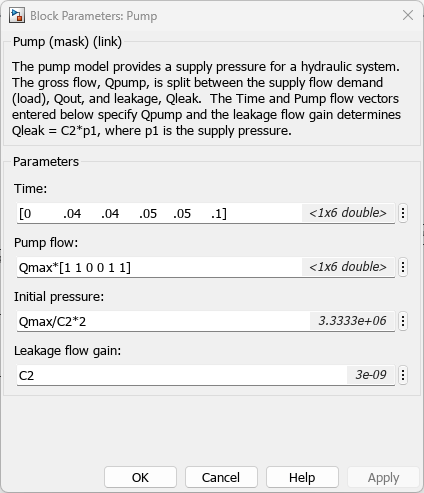Figure 4: Entering pump parameters

'Valve/Cylinder/Piston/Spring Assembly' Subsystem

Right-click the 'Valve/Cylinder/Piston/Spring Assembly' subsystem and select Mask > Look Under Mask to see the Actuator subsystem (see Figure 5). A system of differential-algebraic equations models the cylinder pressurization with the pressure p3, which appears as a derivative in Equation Block 3 and is used as the state (integrator). If we neglect piston mass, the spring force and piston position are direct multiples of p3 and the velocity is a direct multiple of p3's time derivative. This latter relationship forms an algebraic loop around the 'Beta' Gain block. The intermediate pressure p2 is the sum of p3 and the pressure drop due to the flow from the valve to the cylinder (Equation Block 4). This relationship also imposes an algebraic constraint through the control valve and the 1/C1 gain.

The control valve subsystem computes the orifice (Equation Block2). It uses as inputs the upstream and downstream pressures and the variable orifice area. The 'Control Valve Flow' Subsystem computes the signed square root: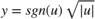Three nonlinear functions are used, two of which are discontinuous. In combination, however, y is a continuous function of u.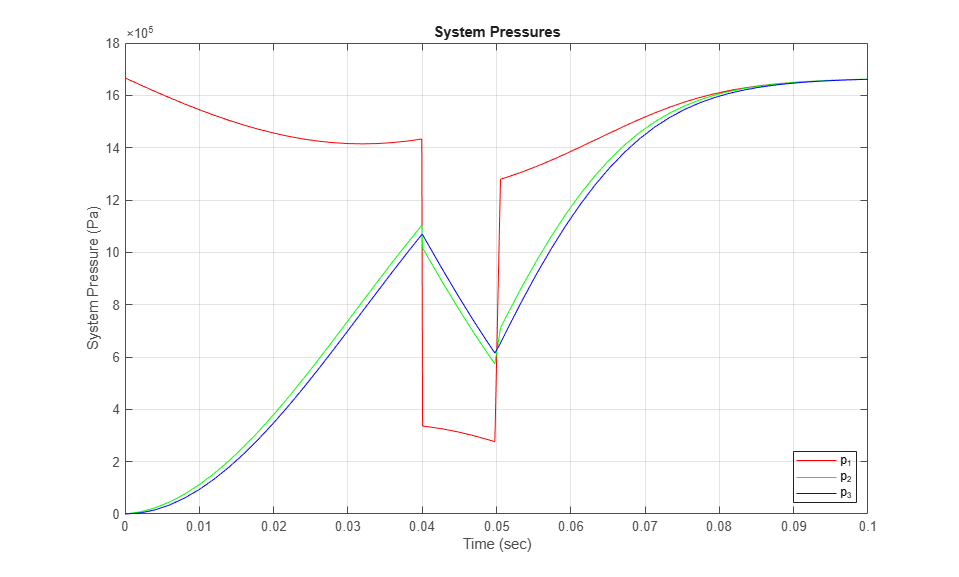Figure 5: The valve/cylinder/piston/spring subsystem

Results

Simulation Parameters

We simulated the model using the following data. The information is loaded from a MAT-file - sldemo_hydcyl_data.mat, which is also used for the other two hydraulic cylinder models. The users can enter data via the Pump and Cylinder Masks shown in Figures 4 and 6.T = [0 0.04 0.04 0.05 0.05 0.1 ] sec

Q = [0.005 0.005 0 0 0.005 0.005] m^3/sec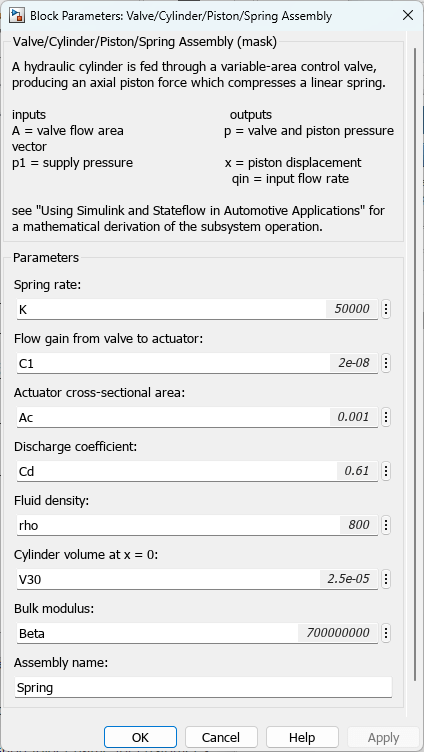Figure 6: Entering valve/cylinder/piston/spring assembly parameters

Plotting Simulation Results

The system initially steps to a pump flow of 0.005 m^3/sec=300 l/min, abruptly steps to zero at t=0.04 sec, then resumes its initial flow rate at t=0.05 sec.

The control valve starts with zero orifice area and ramps to 1e-4 sq.m. during the 0.1 sec simulation time. With the valve closed, all of the pump flow goes to leakage so the initial pump pressure increases to p10 = Q/C2 = 1667 kPa.

As the valve opens, pressures p2 and p3 build up while p1 decreases in response to the load increase as shown in Figure 7. When the pump flow cuts off, the spring and piston act like an accumulator and p3 decreases continuously. Then the flow reverses direction, so p2, though relatively close to p3, falls abruptly. At the pump itself, all of the back-flow leaks and p1 drops radically. The behavior reverses as the flow is restored.

The piston position is directly proportional to p3, where the hydraulic and spring forces balance. Discontinuities in the velocity at 0.04 sec and 0.05 sec indicate negligible mass. The model reaches a steady state when all of the pump flow again goes to leakage, now due to zero pressure drop across the control valve (which means p3 = p2 = p1 = p10).Figure 7: Simulation Results: System PressuresFigure 8: Simulation Results: Hydraulic Cylinder Piston Position

Closing the Model

Close the model and clear generated data.